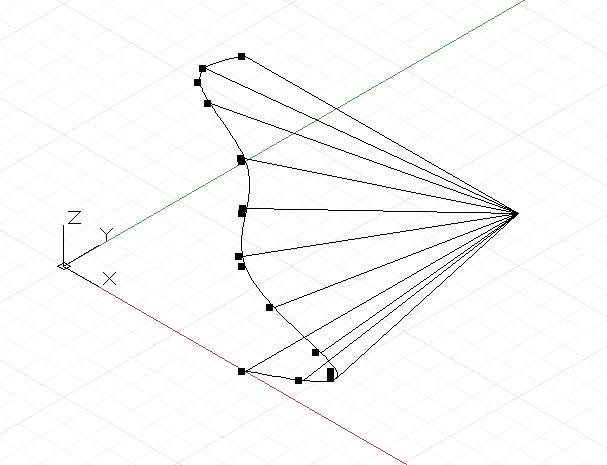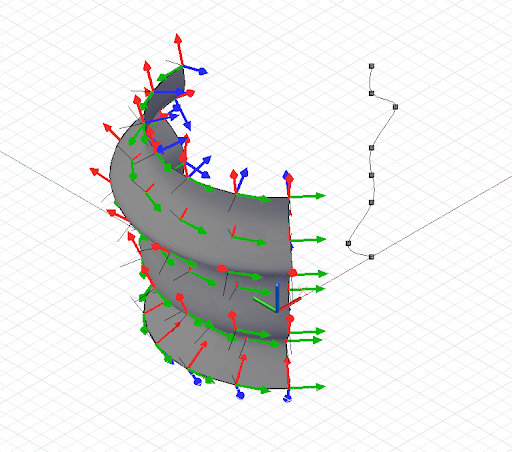Geometric Parameterization

In computational designs, curves and surfaces are frequently used as the underlying scaffold to construct subsequent geometry. In order for this early geometry to be used as a foundation for later geometry, the script must be able to extract qualities such as position and orientation across the entire area of the object. Both curves and surfaces support this extraction, and it is called parameterization.

All of the points on a curve can be thought of as having a unique parameter ranging from 0 to 1. If we were to create a NurbsCurve based off of several control or interpolated points, the first point would have the parameter 0, and the last point would have the parameter 1. It’s impossible to know in advance what the exact parameter is any intermediate point is, which may sound like a severe limitation though is mitigated by a series of utility functions. Surfaces have a similar parameterization as curves, though with two parameters instead of one, called u and v. If we were to create a surface with the following points:

pts = [ [p1, p2, p3],
[p4, p5, p6],
[p7, p8, p9] ];

p1 would have parameter u = 0 v = 0, while p9 would have parameters u = 1 v = 1.

Parameterization isn’t particularly useful when determining points used to generate curves, its main use is to determine the locations if intermediate points generated by NurbsCurve and NurbsSurface constructors.

Curves have a method PointAtParameter, which takes a single double argument between 0 and 1, and returns the Point object at that parameter. For instance, this script finds the Points at parameters 0, .1, .2, .3, .4, .5, .6, .7, .8, .9, and 1:pts = {};
pts = Point.ByCoordinates(4, 0, 0);
pts = Point.ByCoordinates(6, 0, 1);
pts = Point.ByCoordinates(4, 0, 2);
pts = Point.ByCoordinates(4, 0, 3);
pts = Point.ByCoordinates(4, 0, 4);
pts = Point.ByCoordinates(3, 0, 5);
pts = Point.ByCoordinates(4, 0, 6);

crv = NurbsCurve.ByPoints(pts);

pts_at_param = crv.PointAtParameter(0..1..#11);

// draw Lines to help visualize the points
lines = Line.ByStartPointEndPoint(pts_at_param,
Point.ByCoordinates(4, 6, 0));

Similarly, Surfaces have a method PointAtParameter which takes two arguments, the u and v parameter of the generated Point.

While extracting individual points on a curve and surface can be useful, scripts often require knowing the particular geometric characteristics at a parameter, such as what direction the Curve or Surface is facing. The method CoordinateSystemAtParameter finds not only the position but an oriented CoordinateSystem at the parameter of a Curve or Surface. For instance, the following script extracts oriented CoordinateSystems along a revolved Surface, and uses the orientation of the CoordinateSystems to generate lines which are sticking off normal to the surface:pts = {};
pts = Point.ByCoordinates(4, 0, 0);
pts = Point.ByCoordinates(3, 0, 1);
pts = Point.ByCoordinates(4, 0, 2);
pts = Point.ByCoordinates(4, 0, 3);
pts = Point.ByCoordinates(4, 0, 4);
pts = Point.ByCoordinates(5, 0, 5);
pts = Point.ByCoordinates(4, 0, 6);
pts = Point.ByCoordinates(4, 0, 7);

crv = NurbsCurve.ByPoints(pts);

axis_origin = Point.ByCoordinates(0, 0, 0);
axis = Vector.ByCoordinates(0, 0, 1);

surf = Surface.ByRevolve(crv, axis_origin, axis, 90,
140);

cs_array = surf.CoordinateSystemAtParameter(
(0..1..#7)<1>, (0..1..#7)<2>);

def make_line(cs : CoordinateSystem) {
lines_start = cs.Origin;
lines_end = cs.Origin.Translate(cs.ZAxis, -0.75);

return = Line.ByStartPointEndPoint(lines_start,
lines_end);
}

lines = make_line(Flatten(cs_array));

As mentioned earlier, parameterization is not always uniform across the length of a Curve or a Surface, meaning that the parameter 0.5 doesn’t always correspond to the midpoint, and 0.25 doesn’t always correspond to the point one quarter along a curve or surface. To get around this limitation, Curves have an additional set of parameterization commands which allow you to find a point at specific lengths along a Curve.